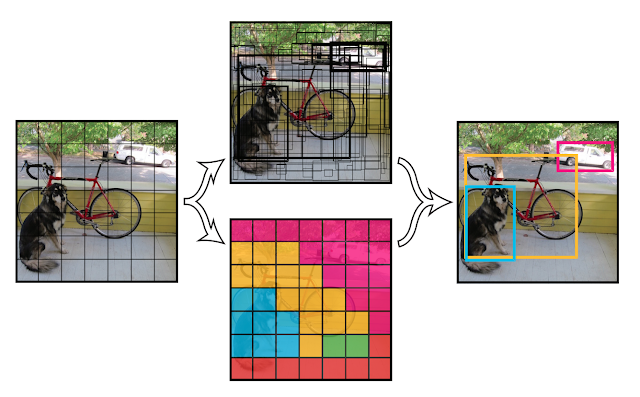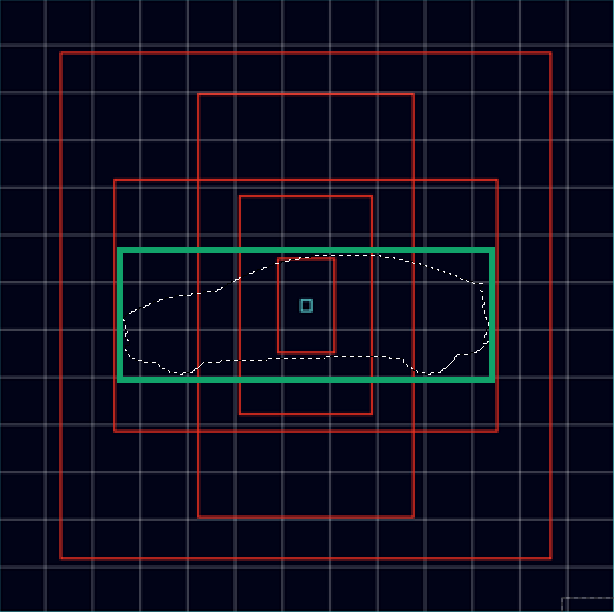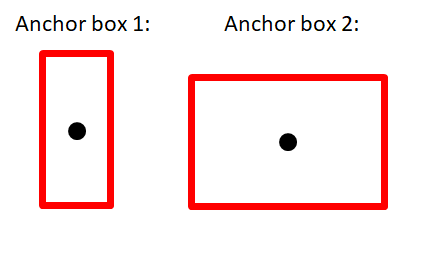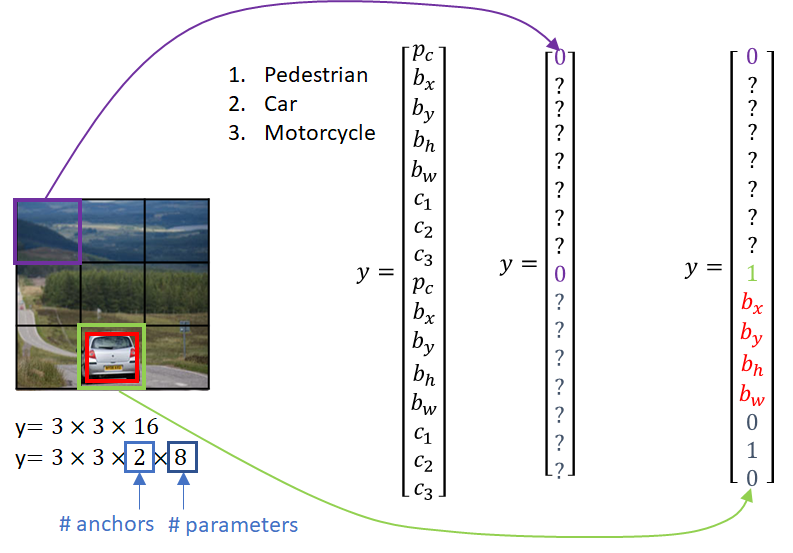## Saturday, 11 January 2020

### Object Detection with YOLO

YOLO (You Only Look Once), together with SSD (Single Shot Detection), OverFeat and some other methods belongs to a family of Object Detection algorithms which are known as "single-shot" object detectors as entire image is taken ("looked") and passed forward through network only once.

YOLO paper ([1506.02640] You Only Look Once: Unified, Real-Time Object Detection) was submitted in June 2015 and revised last time in May 2016.

## Objective

Fast Object Detection.

## Evolution of the Idea

Let's look two families that tried to improve on basic Sliding Window detector and see their strengths and waknesses.

Most advanced Region-proposal detectors (Faster R-CNN):
• RoIs are learned => good bounding box accuracy
• RoIs are further processed separately => low speed

Fully-convolutional approach (OverFeat):
• RoIs are not learned => low bounding box accuracy
• RoIs are processed in one go => high speed

To advance the improvement, let’s take the best of both :
• Learning RoIs
• Processing them in one go

Idea:
• No regions, image as taken as a whole.
• Image passed once (in a “single shot”) through Fully Convolutional NN (FCNN).
• FCNN simultaneously predicts:
• all bounding boxes (regression)
• class probabilities (classification) for each BBox
Benefits:
• Training only a single neural network is required
• Faster inference ⇒ NN can be used for object detection in real-time videos
• Network architecture is lighter ⇒ NN can be deployed on embedded/mobile devices

Implementations: YOLO, SSD, DetectNet

Region-based detectors are all doing two things: proposing potential bounding boxes (RoIs) and then performing classification on them.
After classification, post-processing is used to refine the bounding box, eliminate duplicate detections, and rescore the box based on other objects in the scene. These complex pipelines are slow and hard to optimize because each individual component must be trained separately.

The idea in YOLO/SSD is that take detection completely as regression problem. Rather than doing independent processing for each of these potential regions instead we want to try to treat this like a regression problem and just make all these predictions all at once with a single convolutional network.

A single neural network predicts bounding boxes and class probabilities directly from
full images in one evaluation.YOLO Detection System Image source: Joseph Redmon, Santosh Divvala, Ross Girshick, Ali Farhadi: "You Only Look Once: Unified, Real-Time Object Detection"

Use equidistant grid or predictors which predict set of bounding boxes and apply classification within them.

Create grid of fixed, equidistant detectors: divide image into equal static S*S grid cells.

For each cell neural network predicts:
• B anchor boxes (which can go out of their cells; B is number of ground truth boxes used in labeling; they enable each cell to predict more than 1 object) and for each of them:
• location and size ((x, y) - box center, w - width, h - height). All these variables are scaled to [0, 1] range. x and y are relative to (0, 0) point of the image and w and h are relative to image's total width and height. We can say that image's upper left corner is (0, 0) and lower right corner is (1, 1).
• confidence score (a number between 0 and 1) made of:
• probability that box contains some object (objectness):  P(object)
• IOU (Intersection over Union) - how accurate predicted box matches the ground truth
• (conditional) probability for each class c that this cell contains object of that class (if it contains any object at all): P(c|object)

Neural network output parameters (prediction) is a tensor with these dimensions:
(S*S)*(B*5+C)

YOLO turns object detection into regression problem (in contrast to classification used elsewhere).

Confidence score that given box contains a certain object (class) is:
P(c|object)*P(object)*IOU = P(c)* IOU

Non-max suppression:
• for each class promote only boxes with confidences above the threshold
• object has to be detected by one anchor box - the one with the highest confidence score and Intersection over Union (IoU) with nearby anchors will be taken, others will be discarded (iteratively)

Object is detected by one cell only - the one which contains its centre point. But one cell can predict multiple objects.

Cell predicts B anchor boxes to address cases where multiple objects have centrepoints in one cell.

k-Means clustering used on training sets to determine anchor boxes sizes and number (B).YOLO Model Image source: Joseph Redmon, Santosh Divvala, Ross Girshick, Ali Farhadi: "You Only Look Once: Unified, Real-Time Object Detection"

Example:

7 * 7 grid, 2 anchor boxes (e.g. one horizontal and one vertical) per cell, 20 classes:
(7 * 7) * (2 * 5 + 20) = 1470 outputs (which is not much for typical neural network)

In original YOLO paper the output tensor has dimensions S x S x (B * 5 + C) but in YOLOv2 it was changed to S x S x (B * (5 + C)). (https://leimao.github.io/blog/YOLOs/, https://medium.com/@amrokamal_47691/yolo-yolov2-and-yolov3-all-you-want-to-know-7e3e92dc4899). This is also the output that Andrew Ng is using in his YOLO lecture.Ground truth bounding box has the highest IoU with smaller horizontal anchor. This means that when labeling this image, we'll set confidence score to 1 only for that anchor box while for others it will be 0.

So that's just where we have B base bounding boxes, we have five numbers for each giving our offset and our confidence for that base bounding box and C classification scores for our C categories.

So then we kind of see object detection as this input of an image, output of this three dimensional tensor and you can imagine just training this whole thing with a giant convolutional network.

And that's kind of what these single shot methods do where they just, and again matching the ground truth objects into these potential base boxes becomes a little bit hairy but that's what these methods do.
Ground truth bounding box has the highest IoU with smaller horizontal anchor.
This means that when labeling this image, we'll set confidence score to 1 only for that anchor box while for others it will be 0.

In practice anchor boxes are predetermined. k-means clustering is used on training set to find out what are the most common bounding boxes and they are then grouped in B groups. In the example presented in the image above, we have 5 anchor boxes and they have fixed size. Their size is used for calculating IoU with ground truth bounding boxes for determining to which anchor belongs the object. This information is then used in:
• labelling - we then know where in output y vector we need to place data for particular object
• inference - Non-max suppression (see its description above)

BK: Naturally, objects come in such shapes/positions that can be surrounded by either horizontal or vertical bounding boxes of rectangular shape of various sizes and aspect ratios. We use such "average" anchor boxes when deciding to which of them to assign ground truth box. They are like some kind of a "reference" upon we label training sets. Yolo would also predict such bounding boxes and that's why it's not super accurate.

For each anchor box, system will then not be predicting its dimensions but its offset to the ground truth bounding box. Anchor box with the smallest offset is the one with highest IoU and it will be promoted to the predicted bounding box.

## Network

 Darknet: CNN for the real-time object detection with high accuracy. Single feedforward ConvNet that predicts BBs and class probabilities in a single evaluation Built on GoogleNet which is 22 layers; added 2 convolutional layers and 2 fully connected layers (for inference and regression on the bounding box center coordinates as well as the size and width which can range over the whole image).YOLO Architecture Image source: Joseph Redmon, Santosh Divvala, Ross Girshick, Ali Farhadi: "You Only Look Once: Unified, Real-Time Object Detection"

## Training

Fully supervised.

YOLO trains on full images.

YOLO predicts multiple bounding boxes per grid cell. At training time we only want one bounding box predictor to be responsible for each object. We assign one predictor to be “responsible” for predicting an object based on which prediction has the highest current IOU with the ground truth. This leads to specialization between the bounding box predictors. Each predictor gets better at predicting certain sizes, aspect ratios, or classes of object, improving overall recall. 

Labeling training set:
• k-Means clustering used on training sets to determine anchor box sizes & their number

BK: These B anchor boxes are used for two things:

1) To help placing ground truth bounding box coordinates at the right position (for a specific anchor box) in the label vector. This specific anchor box is the one for which IoU with ground truth box is the highest.

2) To determine at the output which predicted coordinates (or predicted offsets) belong to which anchor box.

Yolo network does not use ever fixed sizes of anchor boxes. It only predicts each anchor box and anchor boxes at the network output will match those in the input ((x, y, w, h) at the specific location in the output vector are predictions learned after observing all quadruplets (x, y, w, h) at the same location in the input label vector...as simple as that, just like any other regression - see the image below).

• Each object in image is assigned to cell that contains object’s midpoint and anchor box (belonging to that cell) with the highest IoU ⇒ cell where centre of the objects falls is responsible for detecting it ⇒ P(classi)=1
• for all cells which do not contain midpoint of any object P(object) == 0 and label is [0, ?,?,?,?] (? means don't care). So for example if we have two objects in the image, only two grid cells will have [1, x, y, w, h] all other will have [0, ?,?,?,?]
• if anchor box size matches the ground truth box ⇔ P(object)=1 ∧ IOU=1

Training set labeling: label vector matches network outputExample of 2 potential anchor boxes.The role of Anchor boxes is to hold the information about the location of potential bounding boxes.Image source: datahacker.rs

Each of these y vectors belongs to a single cell so the complete y vector used in labeling is made of 3 x 3 such small y vectors.

Cells and anchors give spatial information to the regression output. If we don't use anchors, our neural network would be predicting classes for each cell and bounding boxes would be inaccurate as they would be matching exactly the borders of cells.

## Training

For training we use convolutional weights that are pre-trained on Imagenet.
Extensive data augmentation.

## Inference

Runs once on entire image. Very fast.

To enable using video files and streams from network or web cameras: compile it with OpenCV
On Ubuntu install libhighgui-dev and libopencv-dev (sudo apt-get install).

• It makes predictions with a single network evaluation ⇒ huge speed (~45 fps; Fast YOLO 155 fps); 10x faster than Faster R-CNN (which had been developed at the same time as Yolo and 2-step algorithm: region proposal + classification; 7 fps) (Pascal  2007 dataset)
• First CNN-based model which could be used for object detection in real-time videos
• Looks at the whole image at test time ⇒ predictions are informed by global context in the image
• far less likely to predict false detections where nothing exists

• (slightly) less precise localization than Faster R-CNN
• Lower detection performance on smaller objects
• Struggles with unusual aspect ratios
• Performances improved in YOLOv2 (YOLO9000) and YOLOv3

YOLO

YOLOv2

YOLOv3

YOLOv4

YOLOv5

## References:

 Joseph Redmon, Santosh Divvala, Ross Girshick, Ali Farhadi: "You Only Look Once: Unified, Real-Time Object Detection"
 Lecture 11 | Detection and Segmentation - YouTube

Lei Mao's Log Book – Introduction to YOLOs

Understanding YOLO - By

machine learning - How to label training data for YOLO - Stack Overflow

yolo.pdf
YOLO9000.pdf

k-means clustering for anchor boxes - Lars’ Blog

Non-maximum Suppression (NMS) - Towards Data Science

neural network - How is the number of grid cells in YOLO determined? - Data Science Stack Exchange

(18) How can I label an image to train YOLO automatically? - Quora

One-stage object detection

A Practical Guide to Object Detection using the Popular YOLO Framework

A Comprehensive Guide To Object Detection Using YOLO Framework — Part II (Implementing using Python)

#029 CNN Yolo Algorithm | Master Data Science

YOLO, YOLOv2 and YOLOv3: All You want to know - Amro Kamal - Medium

Understanding YOLO and YOLOv2 | Manal El Aidouni

Juan Du: Understanding of Object Detection Based on CNN Family and YOLO

Lecture 11: Detection and Localization

Study of Using Deep Learning Nets for Mark Detection in Space Docking Control Images